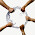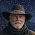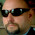## Tuesday, 26 February 2013

### Measuring inductances using an arduino

http://reibot.org/2011/07/19/measuring-inductance/

The technique consists in measuring the resonance frequency of a LC circuit. Since this frequency is:

f = 1.0/(2.0*M_PI*sqrt(L*C))

By measuring f with the arduino and knowing C you can easily get L.

I modified the code so its much more precise, the original code is using 'pulse' to read half an oscillation. My code instead measures one whole oscillation by using an AVR's hw feature called Input Compare, this feaure starts and stops a timer that runs at 16Mhz.

Enjoy!

```//measuring inductance using the higher precision input compare
//based on http://reibot.org/2011/07/19/measuring-inductance/
//capture Flag
volatile uint8_t Flag;
void InitTimer1(void)
{
cli();
//Set Initial Timer value
TCCR1A = 0;
TCCR1B = 0;
// Input Capture Noise Canceller
TCCR1B |= (1 << ICNC1);
//First capture on rising edge
TCCR1B |= (1 << ICES1);
//Start timer without prescaller
TCCR1B &= ~ ((1 << CS12) | (1 << CS11) | (1 << CS10));
TCCR1B |= (1 << CS10);
//Enable input capture and overflow interrupts
TIMSK1 = 0;
TIMSK1 |= (1 << ICIE1) | (1 << TOIE1);
Flag = 0;
sei();
}
volatile uint16_t Capt;
volatile uint16_t T1Ovs2;
//capture ISR
ISR(TIMER1_CAPT_vect)
{
if (Flag == 0)
{
//reset overflows
TCNT1 = 0;
T1Ovs2 = 0;
}
else if (Flag == 1)
{
Capt = ICR1;
//disable capture and overflow interrupts
TIMSK1 &= ~ ((1 << ICIE1) | (1 << TOIE1));
//clear pending interrupts
TIFR1 = (1 << ICF1) | (1 << TOV1);
}
//increment Flag
Flag++;
}
//Overflow ISR
ISR(TIMER1_OVF_vect)
{
T1Ovs2++; //increment overflow counter
}
//13 is the input to the circuit (connects to 150ohm resistor)
//8 is the comparator/op-amp output.
//reibot.org for guide
double pulse, freq, inductance;
//insert capacitance here. Currently using 2uF
double capacitance = 2E-6;
void setup()
{
Serial.begin(115200);
pinMode(8, INPUT);
digitalWrite(8, LOW);
pinMode(13, OUTPUT);
Serial.println("Why hello!");
delay(200);
}
void loop()
{
digitalWrite(13, HIGH);
delay(10); //give some time to charge inductor.
digitalWrite(13, LOW);
delayMicroseconds(15); //make sure resonance is measured
InitTimer1();
delay(100); // wait for ICR to read once cycle
pulse = (T1Ovs2 * 65536 + Capt) / 16.0;
if (pulse > 0.1)
{
freq = 1.E6 / pulse;
inductance = 1. / (capacitance * freq * freq * 4. * 3.14159 * 3.14159);
inductance *= 1E6;
Serial.print("High for uS:");
Serial.print(pulse);
Serial.print("\tfrequency Hz:");
Serial.print(freq);
Serial.print("\tinductance uH:");
Serial.println(inductance);
delay(20);
}
}```

1.With your code are you still using his hardware?

2.can u post the schematic changes required ?

3.This comment has been removed by the author.

4.the readings using your method are pretty stable and never fluctuate, but they are just way way off the mark, seems some calc issues as I can make out. I am using the 741 version and not the 393 version.

Using Author;s original code :
(pretty much accurate, was measuring a 100uH Inductor with a .47 uF cap) Sometimes fluctuates from the base value.

High for uS:22.00 frequency Hz:22727.27 inductance uH:102.92

------------------------------------------------------------------
Using your Code :with same circuit and same everything except the difference of the pin change from 11 to 8
High for uS:4107.25 frequency Hz:121.74 inductance uH:3587073.75

5.Muchas gracias por tu trabajo

6.This code doesn't use the comparator, it just uses input capture. They are not the same.

7.I should thank for the code, but I will not because you do not explain how to measure. I also see that you do not answer any questions that have been asked, so I will not ask in vain.